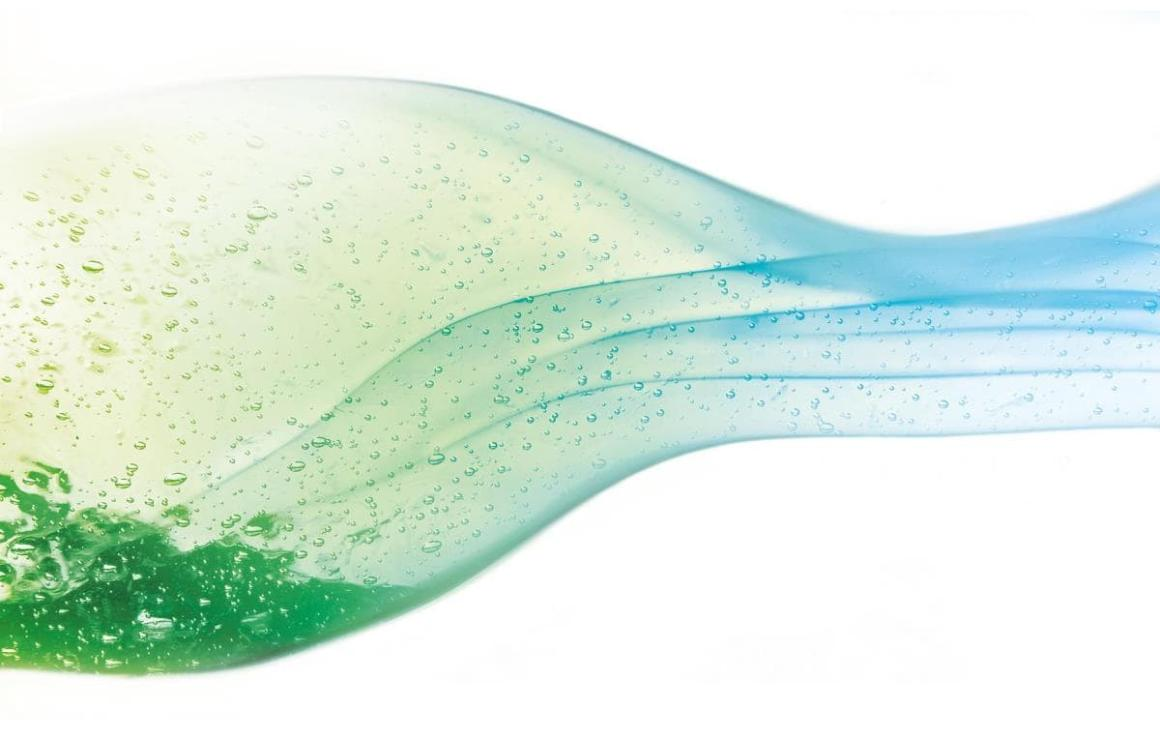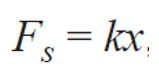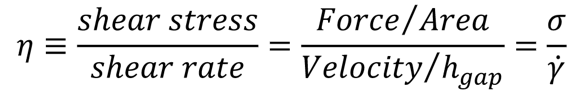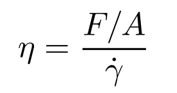# Common Rheology Terms & Concepts

July 6, 2021

In our first installation of our rheology series, we defined rheology, sharing its importance and a few common applications. To review, rheology studies the flow and deformation of substances. Rheology is used to establish the relationship between strains and stresses of substances with complex microstructures, investigate their molecular structure and evaluate their performance.To accurately measure the rheological properties of complex substances, it is important to first have a comprehensive understanding of the terms and equations involved in rheological calculations. In this installation of our rheology blog series, we will be defining important rheological terms and highlighting common rheological concepts.

Rheology measurements are focused on the rheological properties of materials:

• Shear stress τ (mPa): a stress applied parallel to the material cross section on which it acts
• Deformation γ [%]: a change in the dimensions of a body due to an applied external force
• Shear rate γ (1/s): the rate at which progressive shearing deformation is applied to a material

Rheology applies to measurement of deformation and flow of both solids and liquids. When measuring deformation of solids, Hooke’s Law of Elasticity is most often applied, relating stress and strain, or more specifically the force (F) needed to extend or compress a spring by some distance (x).Understanding the force needed to deform a solid is only half of the story. Solids all have a certain elastic modulus, or resistance to being deformed. Modulus of elasticity is a quantity that measures an object or substance's resistance to being deformed non-permanently when a stress is applied to it.A fluids resistance to flow is typically measured as viscosity – the resistance of a fluid to flow. Sir Isacc Newton is most famous in the world of rheology for his description of the flow behavior of fluids using a simple linear relation between shear stress [mPa] and shear rate [1/s]. This is now known as Newton's Law of Viscosity.When measuring these common rheological properties, the multiple factors that must be considered, various measurements you can calculate, and countless equations can seem overwhelming. The first step in easing that feeling of overwhelm is to understand the common rheology terms and concepts.

Newtonian vs. non-Newtonian fluids

Fluids can be both Newtonian (fluids with a simple linear relation between shear stress [mPa] and shear rate [1/s]) and non-Newtonian (viscosity is dependent on shear rate). Most fluids are non-Newtonian, and display either a non-linear relation between shear stress and shear rate, have a yield stress, or viscosity that is dependent on time or deformation history.

To further complicate the idea of Newtonian and non-Newtonian fluids, non-Newtonian behavior of fluids is often broken up into 1 of 3 different categories:

1. Shear thickening is when viscosity of the fluid increases as the shear rate. A common example of shear thickening fluids is a mixture of cornstarch and water.
2. Shear thinning is when viscosity decreases as the shear rate increases. Shear thinning fluids are also known as pseudo-plastics and common examples include paints and blood.
3. Thixotropy is a time-dependent shear thinning property. The most commonly though of thixotropic substance is ketchup.

As noted above, rheology applies to both solids and liquids, however not all materials are solid OR liquid. Some materials exhibit both viscous and elastic characteristics when undergoing deformation. These materials are referred to as viscoelastic materials. Viscous materials, like water, resist shear flow and strain linearly with time when a stress is applied, while elastic materials strain when stretched and immediately return to their original state once the stress is removed. Viscoelastic materials have elements of both of these properties and, as such, exhibit time-dependent strain.

Dynamic vs. Kinematic Viscosity

The most common rheological measurement of fluids is viscosity. There are several types of viscosity, each with their own calculations and units of measurement. The most commonly applied type of viscosity are dynamic viscosity and kinematic viscosity.

Dynamic viscosity

Dynamic viscosity (also known as absolute viscosity) is the measurement of the fluid’s internal resistance to flow. Dynamic viscosity gives you information on the force needed to make the fluid flow at a certain rate. The most commonly used unit for dynamic viscosity is the CGS unit centipoise (cP), however the SI unit for dynamic viscosity (η) is the Pascal-second (Pa-s). Dynamic viscosity can be calculated using the following equation:Another common viscosity measurement is kinematic viscosity. Kinematic viscosity refers to the ratio of dynamic viscosity to density, telling how fast the fluid is moving when a certain force is applied. Kinematic viscosity is often measured in the CGS unit centistokes (cSt), however the SI unit for kinematic viscosity is square meters per second (m2/s). Because kinematic viscosity id a ratio of dynamic viscosity to density, it can be calculated using the following equation:Understanding these common rheological terms and concepts will benefit you in your rheological calculations. In our next installation of this blog series, we will be explaining common rheological calculations as well as model fitting for those calculations. Working with someone who specializes in measuring and understanding these rheological properties can improve formulation, production processes and determine the performance of your products at various conditions - which directly impacts end user/ customer experience. If you would like to learn more about the rheological properties you should be measuring for your applications, contact us to speak with one of our rheology experts today!Written by: Eden Reid, RheoSense Senior Marketing and Sales Operations

## You May Also Like

These Stories on Viscosity Measurements